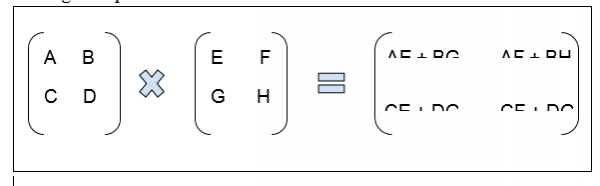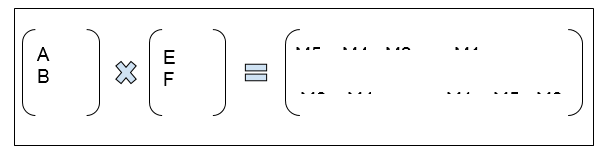# Easy way to remember Strassen's Matrix Equation in C++

It is a matrix multiplication algorithm is based on divide and conquer method. It is used to multiply two matrices of the same size,

Finding multiplication of two matrices−The strassen’s Algorithm reduces overhead for multiplication by simplifying the multiplication.

Here is the multiplication made using the strassen’s Algorithm:M1 = a*(f - h)
M2 = (a + b)*h
M3 = (c + d)*e
M4 = d*(g - e)
M5 = (a + d)*(e + h)
M6 = (b - d)*(g + h)
M7 = (a - c)*(e + f)

This can be easily remembered and the algorithm code can be decoded. For this we have a few rules, first remember these 6 things−

• Use AHED for the first 4 values of M.
• Use Diagonal multiplication for the 5th value of M.
• Use last CR (last col from mat 1 and last row form mat 2) for 6th value of M.
• Use first CR(first col from mat 1 and first row form mat 2) for 7th value of M.
• While considering elements of row add them and in case of columns subtract them.
• The update values after that using adjacent values.

Using these ways we can easily remember the values.